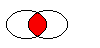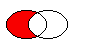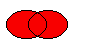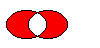# QRegion#

The `QRegion` class specifies a clip region for a painter. More

## Detailed Description#

`QRegion` is used with `setClipRegion()` to limit the paint area to what needs to be painted. There is also a `repaint()` function that takes a `QRegion` parameter. `QRegion` is the best tool for minimizing the amount of screen area to be updated by a repaint.

This class is not suitable for constructing shapes for rendering, especially as outlines. Use `QPainterPath` to create paths and shapes for use with `QPainter` .

`QRegion` is an implicitly shared class.

### Creating and Using Regions#

A region can be created from a rectangle, an ellipse, a polygon or a bitmap. Complex regions may be created by combining simple regions using `united()` , `intersected()` , `subtracted()` , or `xored()` (exclusive or). You can move a region using `translate()` .

You can test whether a region `isEmpty()` or if it `contains()` a `QPoint` or `QRect` . The bounding rectangle can be found with `boundingRect()` .

Iteration over the region (with `begin()` , `end()` , or C++11 ranged-for loops) gives a decomposition of the region into rectangles.

Example of using complex regions:

```def paintEvent(self, arg__0):

r1 = QRegion(QRect(100, 100, 200, 80)
QRegion.Ellipse)
r2 = QRegion(QRect(100, 120, 90, 30))
r3 = r1.intersected(r2) # r3: intersection
painter = QPainter(self)
painter.setClipRegion(r3)
# ...                                  // paint clipped graphics
```
class PySide6.QtGui.QRegion#

PySide6.QtGui.QRegion(bitmap)

PySide6.QtGui.QRegion(pa[, fillRule=Qt.OddEvenFill])

PySide6.QtGui.QRegion(r[, t=QRegion.RegionType.Rectangle])

PySide6.QtGui.QRegion(region)

PySide6.QtGui.QRegion(x, y, w, h[, t=QRegion.RegionType.Rectangle])

Parameters

Constructs an empty region.

Constructs a region from the bitmap `bm`.

The resulting region consists of the pixels in bitmap `bm` that are `color1` , as if each pixel was a 1 by 1 rectangle.

This constructor may create complex regions that will slow down painting when used. Note that drawing masked pixmaps can be done much faster using `setMask()` .

Constructs a polygon region from the point array `a` with the fill rule specified by `fillRule`.

If `fillRule` is `WindingFill` , the polygon region is defined using the winding algorithm; if it is `OddEvenFill` , the odd-even fill algorithm is used.

Warning

This constructor can be used to create complex regions that will slow down painting when used.

Create a region based on the rectangle `r` with region type `t`.

If the rectangle is invalid a null region will be created.

`RegionType`

Constructs a new region which is equal to region `r`.

Constructs a rectangular or elliptic region.

If `t` is `Rectangle`, the region is the filled rectangle (`x`, `y`, `w`, `h`). If `t` is `Ellipse`, the region is the filled ellipse with center at (`x` + `w` / 2, `y` + `h` / 2) and size (`w` ,``h``).

PySide6.QtGui.QRegion.RegionType#

Specifies the shape of the region to be created.

Constant

Description

QRegion.Rectangle

the region covers the entire rectangle.

QRegion.Ellipse

the region is an ellipse inside the rectangle.

PySide6.QtGui.QRegion.__getitem__()#
PySide6.QtGui.QRegion.__len__()#
PySide6.QtGui.QRegion.begin()#
Return type

`PySide6.QtCore.QRect`

Returns a `const_iterator` pointing to the beginning of the range of non-overlapping rectangles that make up the region.

The union of all the rectangles is equal to the original region.

`rbegin()` `cbegin()` `end()`

PySide6.QtGui.QRegion.boundingRect()#
Return type

`PySide6.QtCore.QRect`

Returns the bounding rectangle of this region. An empty region gives a rectangle that is `isNull()` .

PySide6.QtGui.QRegion.cbegin()#
Return type

`PySide6.QtCore.QRect`

Same as `begin()` .

PySide6.QtGui.QRegion.cend()#
Return type

`PySide6.QtCore.QRect`

Same as `end()` .

PySide6.QtGui.QRegion.contains(p)#
Parameters
Return type

bool

Returns `true` if the region contains the point `p`; otherwise returns `false`.

PySide6.QtGui.QRegion.contains(r)
Parameters
Return type

bool

Returns `true` if the region overlaps the rectangle `r`; otherwise returns `false`.

PySide6.QtGui.QRegion.end()#
Return type

`PySide6.QtCore.QRect`

Returns a `const_iterator` pointing to one past the end of non-overlapping rectangles that make up the region.

The union of all the rectangles is equal to the original region.

`rend()` `cend()` `begin()`

PySide6.QtGui.QRegion.intersected(r)#
Parameters
Return type

`PySide6.QtGui.QRegion`

Returns a region which is the intersection of this region and the given `rect`.

PySide6.QtGui.QRegion.intersected(r)
Parameters
Return type

`PySide6.QtGui.QRegion`

Returns a region which is the intersection of this region and `r`.The figure shows the intersection of two elliptical regions.

PySide6.QtGui.QRegion.intersects(r)#
Parameters
Return type

bool

Returns `true` if this region intersects with `rect`, otherwise returns `false`.

PySide6.QtGui.QRegion.intersects(r)
Parameters
Return type

bool

Returns `true` if this region intersects with `region`, otherwise returns `false`.

PySide6.QtGui.QRegion.isEmpty()#
Return type

bool

Returns `true` if the region is empty; otherwise returns `false`. An empty region is a region that contains no points.

Example:

```r1 = QRegion(10, 10, 20, 20)
r1.isEmpty() # false
r3 = QRegion()
r3.isEmpty() # true
r2 = QRegion(40, 40, 20, 20)
r3 = r1.intersected(r2) # r3: intersection of r1 and r2
r3.isEmpty() # true
r3 = r1.united(r2) # r3: union of r1 and r2
r3.isEmpty() # false
```
PySide6.QtGui.QRegion.isNull()#
Return type

bool

Returns `true` if the region is empty; otherwise returns `false`. An empty region is a region that contains no points. This function is the same as `isEmpty`

PySide6.QtGui.QRegion.__ne__(r)#
Parameters
Return type

bool

Returns `true` if this region is different from the `other` region; otherwise returns `false`.

PySide6.QtGui.QRegion.__and__(r)#
Parameters
Return type

`PySide6.QtGui.QRegion`

Applies the `intersected()` function to this region and `r`. `r1&r2` is equivalent to `r1.intersected(r2)`.

PySide6.QtGui.QRegion.__and__(r)
Parameters
Return type

`PySide6.QtGui.QRegion`

PySide6.QtGui.QRegion.__mul__(m)#
Parameters
Return type

`PySide6.QtGui.QRegion`

Parameters
Return type

`PySide6.QtGui.QRegion`

Parameters
Return type

`PySide6.QtGui.QRegion`

Applies the `united()` function to this region and `r`. `r1+r2` is equivalent to `r1.united(r2)`.

`united()` `operator|()`

Parameters
Return type

`PySide6.QtGui.QRegion`

Returns a region that is the union of this region with the specified `rect`.

Parameters
Return type

`PySide6.QtGui.QRegion`

Applies the `united()` function to this region and `r` and assigns the result to this region. `r1+=r2` is equivalent to `r1 = r1.united(r2)`.

PySide6.QtGui.QRegion.__sub__(r)#
Parameters
Return type

`PySide6.QtGui.QRegion`

Applies the `subtracted()` function to this region and `r`. `r1-r2` is equivalent to `r1.subtracted(r2)`.

PySide6.QtGui.QRegion.__isub__(r)#
Parameters
Return type

`PySide6.QtGui.QRegion`

Applies the `subtracted()` function to this region and `r` and assigns the result to this region. `r1-=r2` is equivalent to `r1 = r1.subtracted(r2)`.

PySide6.QtGui.QRegion.__eq__(r)#
Parameters
Return type

bool

Returns `true` if the region is equal to `r`; otherwise returns false.

PySide6.QtGui.QRegion.__xor__(r)#
Parameters
Return type

`PySide6.QtGui.QRegion`

Applies the `xored()` function to this region and `r`. `r1^r2` is equivalent to `r1.xored(r2)`.

PySide6.QtGui.QRegion.__ixor__(r)#
Parameters
Return type

`PySide6.QtGui.QRegion`

Applies the `xored()` function to this region and `r` and assigns the result to this region. `r1^=r2` is equivalent to `r1 = r1.xored(r2)`.

PySide6.QtGui.QRegion.__or__(r)#
Parameters
Return type

`PySide6.QtGui.QRegion`

Applies the `united()` function to this region and `r`. `r1|r2` is equivalent to `r1.united(r2)`.

`united()` `operator+()`

PySide6.QtGui.QRegion.__ior__(r)#
Parameters
Return type

`PySide6.QtGui.QRegion`

Applies the `united()` function to this region and `r` and assigns the result to this region. `r1|=r2` is equivalent to `r1 = r1.united(r2)`.

PySide6.QtGui.QRegion.rectCount()#
Return type

int

Returns the number of rectangles that this region is composed of. Same as `end() - begin()`.

PySide6.QtGui.QRegion.setRects(rect, num)#
Parameters
• num – int

Sets the region using the array of rectangles specified by `rects` and `number`. The rectangles must be optimally Y-X sorted and follow these restrictions:

• The rectangles must not intersect.

• All rectangles with a given top coordinate must have the same height.

• No two rectangles may abut horizontally (they should be combined into a single wider rectangle in that case).

• The rectangles must be sorted in ascending order, with Y as the major sort key and X as the minor sort key.

PySide6.QtGui.QRegion.subtracted(r)#
Parameters
Return type

`PySide6.QtGui.QRegion`

Returns a region which is `r` subtracted from this region.The figure shows the result when the ellipse on the right is subtracted from the ellipse on the left (`left - right`).

PySide6.QtGui.QRegion.swap(other)#
Parameters

Swaps region `other` with this region. This operation is very fast and never fails.

PySide6.QtGui.QRegion.translate(p)#
Parameters

Translates the region `point` .x() along the x axis and `point` .y() along the y axis, relative to the current position. Positive values move the region to the right and down.

Translates to the given `point`.

PySide6.QtGui.QRegion.translate(dx, dy)
Parameters
• dx – int

• dy – int

Translates (moves) the region `dx` along the X axis and `dy` along the Y axis.

PySide6.QtGui.QRegion.translated(p)#
Parameters
Return type

`PySide6.QtGui.QRegion`

Returns a copy of the regtion that is translated `p` .x() along the x axis and `p` .y() along the y axis, relative to the current position. Positive values move the rectangle to the right and down.

PySide6.QtGui.QRegion.translated(dx, dy)
Parameters
• dx – int

• dy – int

Return type

`PySide6.QtGui.QRegion`

Returns a copy of the region that is translated `dx` along the x axis and `dy` along the y axis, relative to the current position. Positive values move the region to the right and down.

PySide6.QtGui.QRegion.united(r)#
Parameters
Return type

`PySide6.QtGui.QRegion`

Returns a region which is the union of this region and the given `rect`.

PySide6.QtGui.QRegion.united(r)
Parameters
Return type

`PySide6.QtGui.QRegion`

Returns a region which is the union of this region and `r`.The figure shows the union of two elliptical regions.

PySide6.QtGui.QRegion.xored(r)#
Parameters
Return type

`PySide6.QtGui.QRegion`

Returns a region which is the exclusive or (XOR) of this region and `r`.The figure shows the exclusive or of two elliptical regions.